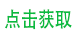# django 组装表名查询数据（动态表名、组合表名）

## 了解 getattr 函数

### 描述

getattr() 函数用于返回一个对象属性值。

### 语法

getattr 语法：

`getattr(object, name[, default])`

### 参数

• object -- 对象。
• name -- 字符串，对象属性。
• default -- 默认返回值，如果不提供该参数，在没有对应属性时，将触发 AttributeError。

## 组装表名查询

``````import myapp.models

def test(requset):
user_db_name = "user_%s" % request.user.name  # 组装表名
user_db = getattr(myapp.models, user_db_name)
user_data = user_db.objects.all()

rerurn "..."``````

# 一个模型动态创建的多个 db_table

``````def get_log_model(prefix):
table_name = 'log_%s' % str(prefix)

LOG_LEVELS = (
(0, 'DEBUG'),
(10, 'INFO'),
(20, 'WARNING'),
)

class LogMetaclass(models.base.ModelBase):
def __new__(cls, name, bases, attrs):
name += '_' + prefix  # 这是Model的name.
return models.base.ModelBase.__new__(cls, name, bases, attrs)

class Log(models.Model):
__metaclass__ = LogMetaclass
level = models.IntegerField(choices=LOG_LEVELS)
msg = models.TextField()

@staticmethod
def is_exists():
return table_name in connection.introspection.table_names()

class Meta:
db_table = table_name

return Log``````

``````print cls.__name__
Log_20181211
print cls._meta.db_table
log_20181211``````

``````def index(request):
today = date.today().strftime("%Y%m%d")

# RuntimeWarning: Model '__main__.logclasslog_' was already registered.
# most notably with related models.
# 如上述警告所述, Django 不建议重复加载 Model 的定义.
# 作为 demo 可以直接通过get_log_model获取，无视警告.
# 所以这里先通过 all_models 获取已经注册的 Model,
# 如果获取不到， 再生成新的模型.
try:
cls = apps.get_model('__main__', 'Log_%s' % today)
except LookupError:
cls = get_log_model(today)

if not cls.is_exists():
with connection.schema_editor() as schema_editor:
schema_editor.create_model(cls)

log = cls(level=10, msg="Hello")
log.save()

return HttpResponse('<h1>%s</h1>' % cls._meta.db_table)``````

^_^